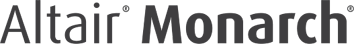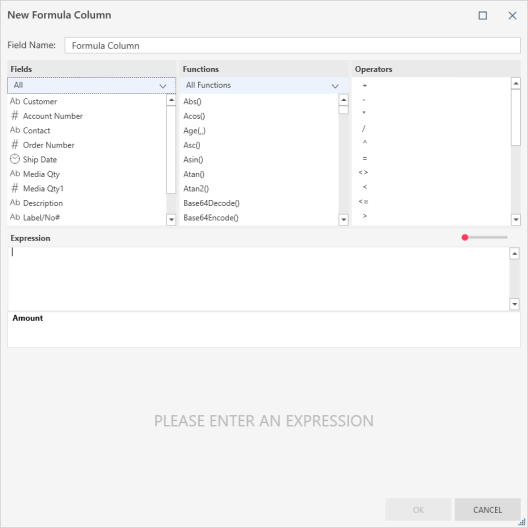# Create Calculated Field

### Description

Creates a new column based on a calculation.

Follow these steps to create a calculated field:

1. Right+Click on a numeric field and select Compute > Create Calculated Field from the context menu. The Create Calculated Field dialog displays:1. Define the calculated field by selecting the calculation components. You can include columns, numbers, and operators:1. Note that:

• Only numeric fields are included in the columns list.

• As you define the formula a sample of the output is displayed in the preview area at the bottom of the dialog box.

1. Select Enter to add the calculation as a new column.

2. Rename the column.

### INPUT/Output DATA TYPE

 Input numeric Output numeric

### example

In this example, the first column (Amount) is the original column. Discount is a calculated field that shows the 10% discount value. It uses the following simple formula: Amount * .10: